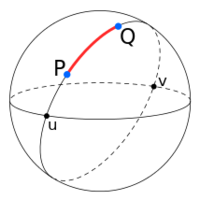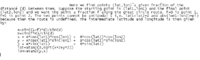# Spherical Trig question

#### markraz

##### Full MemberHi, I'm trying to find the equation of a path on a sphere sort of like this red curve between P and Q above.
I think this solution below can calculate this curve. My question is, what exactly does 'd' represent? Also does the 'f'
variable behave similar 't' in a parametric equation?

Any help will be much appreciated, thanks in advancesource:

#### LCKurtz

##### Full Member
Apparently $$\displaystyle d$$ is a given, or previously calculated great circle distance between the two points. $$\displaystyle f$$ is a parameter between 0 and 1 where the point moves along the curve from P to Q as $$\displaystyle f$$ moves from 0 to 1. So, yes, $$\displaystyle f$$ is like a parameter $$\displaystyle t$$.